# Standard #: MAFS.4.NF.2.3 (Archived Standard)

This document was generated on CPALMS - www.cpalms.org

Understand a fraction a/b with a > 1 as a sum of fractions 1/b.
1. Understand addition and subtraction of fractions as joining and separating parts referring to the same whole.
2. Decompose a fraction into a sum of fractions with the same denominator in more than one way, recording each decomposition by an equation. Justify decompositions, e.g., by using a visual fraction model. Examples: 3/8 = 1/8 + 1/8 + 1/8 ; 3/8 = 1/8 + 2/8 ; 2 1/8 = 1 + 1 + 1/8 = 8/8 + 8/8 + 1/8.
3. Add and subtract mixed numbers with like denominators, e.g., by replacing each mixed number with an equivalent fraction, and/or by using properties of operations and the relationship between addition and subtraction.
4. Solve word problems involving addition and subtraction of fractions referring to the same whole and having like denominators, e.g., by using visual fraction models and equations to represent the problem.

### Clarifications

Examples of Opportunities for In-Depth Focus

This standard represents an important step in the multi-grade progression for addition and subtraction of fractions. Students extend their prior understanding of addition and subtraction to add and subtract fractions with like denominators by thinking of adding or subtracting so many unit fractions.

### General Information

Subject Area: Mathematics
Domain-Subdomain: Number and Operations - Fractions
Cluster: Build fractions from unit fractions by applying and extending previous understandings of operations on whole numbers. (Major Cluster) -

Clusters should not be sorted from Major to Supporting and then taught in that order. To do so would strip the coherence of the mathematical ideas and miss the opportunity to enhance the major work of the grade with the supporting clusters.

Date of Last Rating: 02/14
Status: State Board Approved - Archived
Assessed: Yes

### Test Item Specifications

N/A

Assessment Limits :
Denominators of given fractions are limited to: 2, 3, 4, 5, 6, 8, 10, 12, 100. Mixed numbers and fractions must contain like denominators. Items must reference the same whole. Visual fraction models are limited to circular models, rectangular models, and number line models.
Calculator :

No

Context :

Allowable. Required for MAFS.4.NF.2.3d

### Sample Test Items (5)

 Test Item # Question Difficulty Type Sample Item 1 What is the value of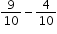? N/A EE: Equation Editor Sample Item 2 What is the value of the following expression?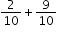N/A MC: Multiple Choice Sample Item 3 Sue had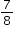of a cup of flour. She used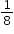of a cup.How much flour, in cups, does Sue have left? N/A EE: Equation Editor Sample Item 4 What is the sum ofand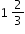? N/A EE: Equation Editor Sample Item 5 Select all the equations that show different ways to represent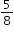. N/A MS: Multiselect

#### Related Courses

 Course Number1111 Course Title222 5012060: Mathematics - Grade Four (Specifically in versions: 2014 - 2015, 2015 - 2022, 2022 and beyond (current)) 7712050: Access Mathematics Grade 4 (Specifically in versions: 2014 - 2015, 2015 - 2018, 2018 - 2022, 2022 and beyond (current)) 5012065: Grade 4 Accelerated Mathematics (Specifically in versions: 2019 - 2022, 2022 and beyond (current)) 5012015: Foundational Skills in Mathematics 3-5 (Specifically in versions: 2019 - 2022, 2022 and beyond (current))

#### Educational Game

 Name Description Fraction Quiz Test your fraction skills by answering questions on this site. This quiz asks you to simplify fractions, convert fractions to decimals and percentages, and answer algebra questions involving fractions. You can even choose difficulty level, question types, and time limit.

#### Formative Assessments

 Name Description Adding and Subtracting Mixed Numbers Students are given pairs of mixed numbers to either add or subtract. Fraction Word Problems Students are asked to solve a word problem that involves subtracting fractions with like denominators. Students then analyze a word problem involving subtraction of unlike unit quantities. Decomposing Three-Fifths Students are asked to use a visual fraction model to decompose three-fifths in two different ways. Anna Marie and the Pizza Students are asked to solve a word problem that involves adding fractions with like denominators. Students then analyze a word problem involving addition of unlike unit quantities.

#### Original Student Tutorial

 Name Description The Leftover Dessert Dilemma Learn how to decompose a fraction into a sum of fractions with common denominators with this interactive tutorial.

#### Tutorials

 Name Description What Fraction of Spider Eyes are Looking at Me? This Khan Academy video uses authentic pictures to present addition of two fractions with common denominators. Figuring Out How Much of a Pizza is Left This Khan Academy video solves two word problems using visual fraction models. Comparing Fractions This tutorial for student audiences will assist learners with a further understanding that fractions are a way of showing part of a whole. Yet some fractions are larger than others. So this tutorial will help to refresh the understanding for the comparison of fractions. Students will be able to navigate the teaching portion of the tutorial at their own pace and test their understanding after each step of the lesson with a "Try This" section. The "Try This" section will monitor students answers and self-check by a right answer turning orange and a wrong answer dissolving. Adding Fractions In this web-based tutorial, students learn procedures for adding fractions with like and unlike denominators. The tutorial includes visual representations of the problems using pizzas, animations of the algorithm, and links to related lessons, worksheets, and practice problems.

#### Virtual Manipulative

 Name Description Fraction Game This virtual manipulative allows individual students to work with fraction relationships. (There is also a link to a two-player version.)

#### Original Student Tutorial

 Name Description The Leftover Dessert Dilemma: Learn how to decompose a fraction into a sum of fractions with common denominators with this interactive tutorial.

#### Educational Game

 Name Description Fraction Quiz: Test your fraction skills by answering questions on this site. This quiz asks you to simplify fractions, convert fractions to decimals and percentages, and answer algebra questions involving fractions. You can even choose difficulty level, question types, and time limit.

#### Tutorials

 Name Description What Fraction of Spider Eyes are Looking at Me?: This Khan Academy video uses authentic pictures to present addition of two fractions with common denominators. Figuring Out How Much of a Pizza is Left: This Khan Academy video solves two word problems using visual fraction models. Comparing Fractions: This tutorial for student audiences will assist learners with a further understanding that fractions are a way of showing part of a whole. Yet some fractions are larger than others. So this tutorial will help to refresh the understanding for the comparison of fractions. Students will be able to navigate the teaching portion of the tutorial at their own pace and test their understanding after each step of the lesson with a "Try This" section. The "Try This" section will monitor students answers and self-check by a right answer turning orange and a wrong answer dissolving.

#### Virtual Manipulative

 Name Description Fraction Game: This virtual manipulative allows individual students to work with fraction relationships. (There is also a link to a two-player version.)